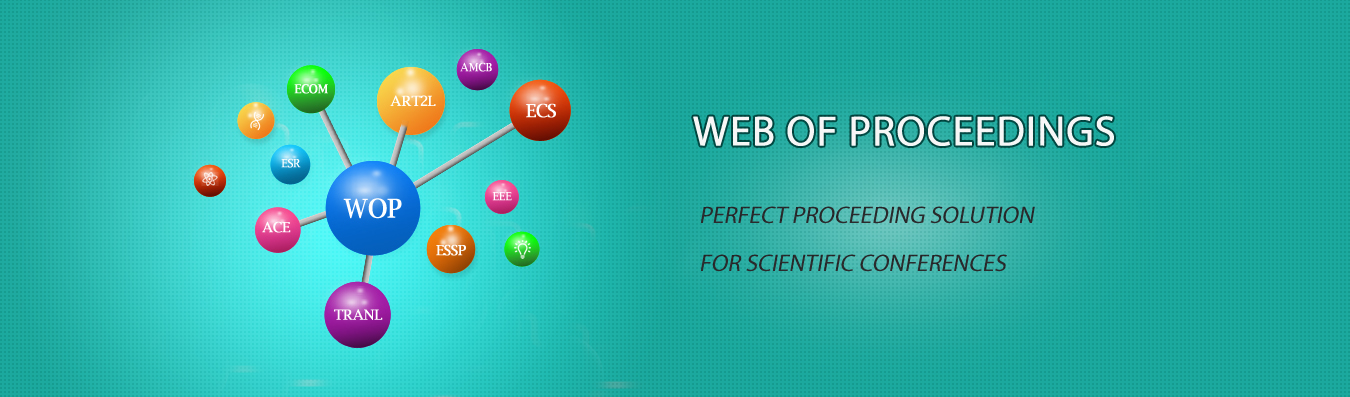The best way to conference proceedings by Francis Academic Press### Research on Parameterization of spacecraft trajectory collision probability based on Covariance Analysis

DOI: 10.25236/isaiee.2020.028

#### Author(s)

Shan Xiao, Zhexin Jiang, Xuan Ding

Shan Xiao

#### Abstract

In order to avoid collision between on-orbit spacecraft and other space targets, when necessary, spacecraft need to take active orbit maneuver to avoid collision risk. With the increase of human space missions and the emergence of a large number of rocket arrows and their debris, invalid satellites and other space debris, the collision probability between space targets is increasing. At the same time, before the daily orbit adjustment or orbit maintenance of the spacecraft, it is also necessary to carry out collision early warning analysis to avoid collision during or after orbit control. To solve this kind of problem, it is necessary to study the influence of orbit control error on collision probability. In this paper, firstly, the propagation model of orbit control error is established based on the description function method of covariance analysis. Secondly, on the basis of the existing calculation methods of two-dimensional collision probability, the calculation method of three-dimensional collision probability of space target under nonlinear relative motion is further given. The calculation accuracy of collision probability is improved. Finally, the influence of orbit control error on collision probability is discussed. The simulation verification of the nonlinear method through specific collision events shows that the collision probability will increase with the increase of the influence of orbit control error on the propagation of position and velocity error, in which the introduction of thrust azimuth deviation has the greatest influence on the collision probability. The introduction of thrust magnitude deviation has the second influence on the collision probability, and the introduction of thrust high and low angle deviation has the least influence on the collision probability.

#### Keywords

Nonlinear system, Track control error, the probability of collision, Covariance analysis description function method